You are here:

# Technological Arts Inc.

## SBASIC Manual - Page 24

Article Index
SBASIC Manual
Page 2
Page 3
Page 4
Page 5
Page 6
Page 7
Page 8
Page 9
Page 10
Page 11
Page 12
Page 13
Page 14
Page 15
Page 16
Page 17
Page 18
Page 19
Page 20
Page 21
Page 22
Page 23
Page 24
Page 25
Page 26
Page 27
Page 28
Page 29
Page 30
Page 31
Page 32
Page 33
Page 34
Page 35
Page 36
Page 37
Page 38
Page 39
Page 40
Page 41
Page 42
Page 43
Page 44
Page 45
Page 46
Page 47
Page 48
Page 49
Page 50
Page 51
Page 52
Page 53
Page 54
Page 55
Page 56
Page 57
Page 58
Page 59
Page 60
Index
All Pages

SBasic User's Manual     SBasic Version 2.7             Page 24
Printed:  December 5, 1999
Operators

SBasic supports all of the common arithmetic operators:

= (equal-sign) sets the value of a variable to the result of a
calculation.  This is the traditional assignment operator.  All
assignments store a 16-bit value into a variable.

- (minus-sign) performs 16-bit subtraction.  It also acts as the unary
negation operator.

~ (tilde) performs 16-bit one's complement.  This is logically
identical to N XOR \$ffff.

* (asterisk) multiplies two 16-bit values, yielding a 16-bit product.

Run-time support for SBasic's multiplication operator on a 68hc11 MCU
relies on a library file, included with the SBasic distribution.  This
file is automatically added to the assembler source file created by
SBasic, whenever your program invokes the multiplication operator.

Note that this library file is only included if your code uses a
multiply operation.  You can create smaller executables by eliminating
any use of the multiplication operator, if appropriate.

For the 68hc12 MCU, multiplication is done with inline assembly using
the EMUL opcode, and executes much faster than it would on a 68hc11.

/ (forward-slash) divides a 16-bit dividend by a 16-bit divisor,
yielding a 16-bit quotient; the remainder is lost.

MOD (modulus) divides a 16-bit dividend by a 16-bit divisor, yielding
a 16-bit remainder; the quotient is lost.

Examples:

alpha = foo + bar        ' adds two variables
beta = gamma - \$1234     ' subtracts a hex constant
var1 = 3 * var2          ' multiplies a variable
c = delta / 88           ' divides a variable by a constant
m = gamma MOD 10         ' takes the modulus function

SBasic also supports most of the common Boolean operators:

AND performs the logical AND of two 16-bit values.

OR performs the logical inclusive-OR of two 16-bit values.

XOR performs the logical exclusive-OR of two 16-bit values.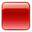Subscribe to our newsletter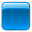Returns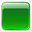Connector Options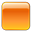Distributors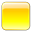FAQ